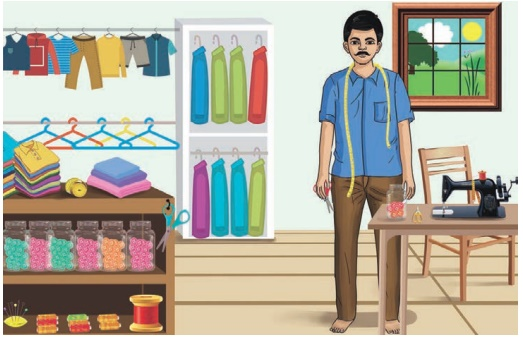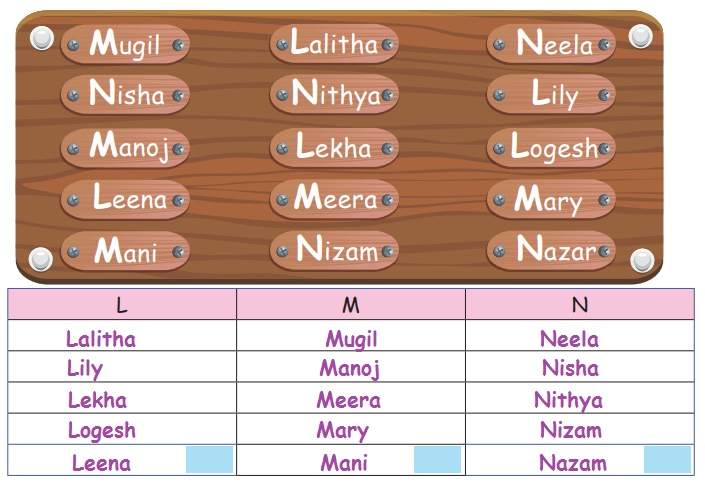Home | | Maths 2nd Std | Grouping

# Grouping

Teacher Note: Teacher can explain the situation and ask a few questions like the following to familiarize the things on grouping of objects * What are the things you see in the picture? How many scissors are there? * How many bottles of buttons are there ?

Grouping

Travel Through

In a tailor shopTeacher Note:  Teacher can explain the situation and ask a few questions like the following to familiarize the things on grouping of objects

* What are the things you see in the picture? How many scissors are there?

* How many bottles of buttons are there ?

Learn

Group the words that begin with the same letter and count them.Words that begin with A,B and C are as following.Practice

Group the words given below by their starting letter, count and write them.Activity

Let us classify and count* Take the materials such as beads, neem seeds, tamarind seeds, pebbles and mix them in a tray.

* Ask the students to group them.

* Tell the students to count and say the number.

Learn

Grouping ones

10 ones make 1 ten.Practice

Group the objects in tens and ones and write the number.Tags : Numbers | Term 1 Chapter 2 | 2nd Maths , 2nd Maths : Term 1 Unit 2 : Numbers
Study Material, Lecturing Notes, Assignment, Reference, Wiki description explanation, brief detail
2nd Maths : Term 1 Unit 2 : Numbers : Grouping | Numbers | Term 1 Chapter 2 | 2nd Maths